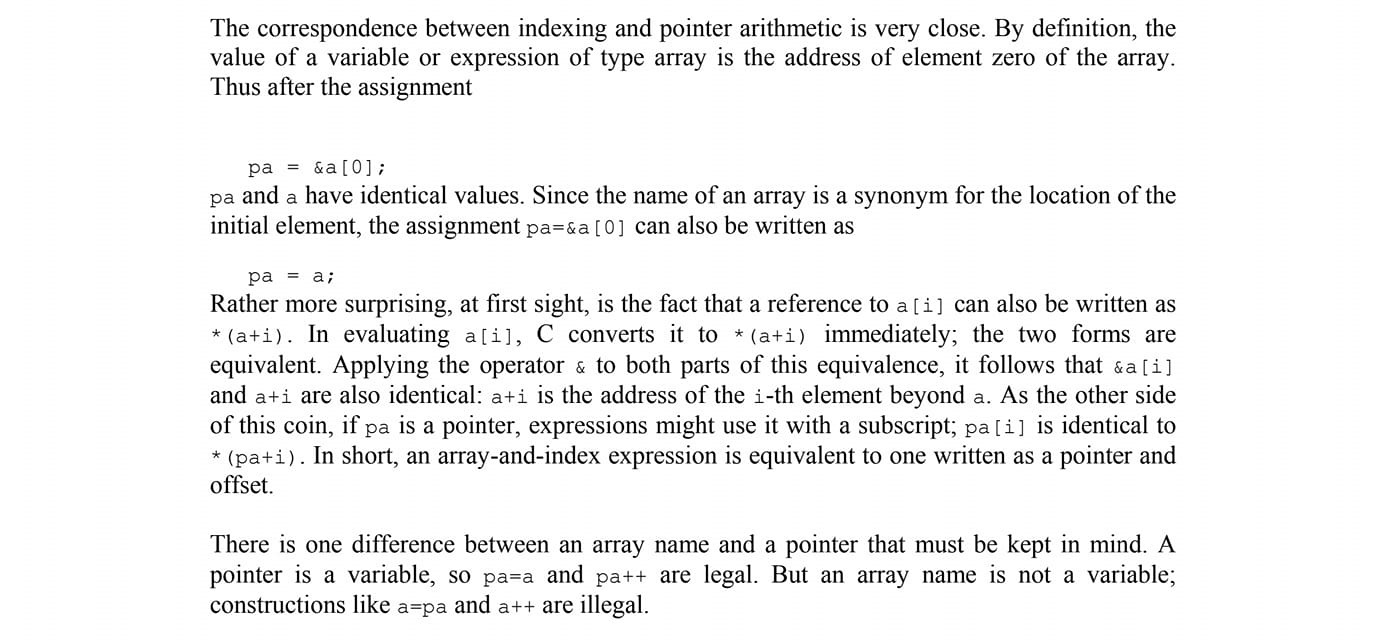# 首先、來公布答案 XD

Array 和 Pointer 是兩種概念，完全不同的東西

# 誤解：Array 就是 Pointer

Pointer 的下標運算子本質上是一個 base address 加上一個 offset

1. 你要取得 array 的 element 你必須使用下標運算子
2. 下標運算子的使用語法上 array[i] 中的 變數名稱 array 似乎可以象徵 base address, 而理所當然的 i 就是 offset
3. 相同型別的 array 可以直接 assign 給 pointer
4. 所以 array 就是 pointer

array 的存取確實是用 base address from offset

# 值(value)

lvalue 的全名是什麼呢？
left hand side value?

(這裡先不討論 C++11 以後的 glvalue, xvalue, ... 的模型)

# &array (address of an array)

``````int n;
std::cout << &n << std::endl;
``````

``````int a;
std::cout << &a << std::endl;
``````

array 的 value 其實就是 elements 構成的儲存空間

``````#include <cstdio>
int main() {
int n = {};
int* pn = n;
printf("%d\n", pn == (void*)n); // output 1
printf("%d\n", pn == (void*)&n); // output 1
printf("%d\n", &(n) == (void*)&n); // output 1
printf("%d\n", &(n) == (void*)n); // output 1
return 0;
}
``````

# 附錄：怎麼從 assembly 看這件事

``````#include <cstdio>
int main() {
int a = 0;
int n = {5, 1, 2};
printf("%d\n", n);
printf("%d\n", a);
return 0;
}
``````

``````.LC0:
.string "%d\n"
main:
pushq   %rbp
movq    %rsp, %rbp
subq    \$16, %rsp
movl    \$0, -4(%rbp)
movl    \$5, -16(%rbp)
movl    \$1, -12(%rbp)
movl    \$2, -8(%rbp)
movl    -12(%rbp), %eax
movl    %eax, %esi
movl    \$.LC0, %edi
movl    \$0, %eax
call    printf
movl    -4(%rbp), %eax
movl    %eax, %esi
movl    \$.LC0, %edi
movl    \$0, %eax
call    printf
movl    \$0, %eax
leave
ret
``````

``````movl    \$5, -16(%rbp)
movl    \$1, -12(%rbp)
movl    \$2, -8(%rbp)
``````

``````movl    -12(%rbp), %eax
movl    %eax, %esi
movl    \$.LC0, %edi
movl    \$0, %eax
call    printf
``````

# 2021/6/28 更新 - The C programming language 書中的描述Thus after the assignment pa = &a; pa and a have identical value.

6.3.2.1 - 3
Except when it is the operand of the sizeof operator or the unary & operator, or is a
string literal used to initialize an array, an expression that has type ‘‘array of type’’ is
converted to an expression with type ‘‘pointer to type’’ that points to the initial element of
the array object and is not an lvalue.

6.5.1 - 2
An identifier is a primary expression, provided it has been declared as designating an
object (in which case it is an lvalue) or a function (in which case it is a function
designator).

lvalue 乃至於 Mordern C++ 提到 glvalue 定義的範圍遠遠不只如此。

### 回到書中的描述

(當然同時我也會告訴他這是不同的東西，僅僅是便於理解）

# reference

#C #C++ #assembly #Computer Science #Compiler